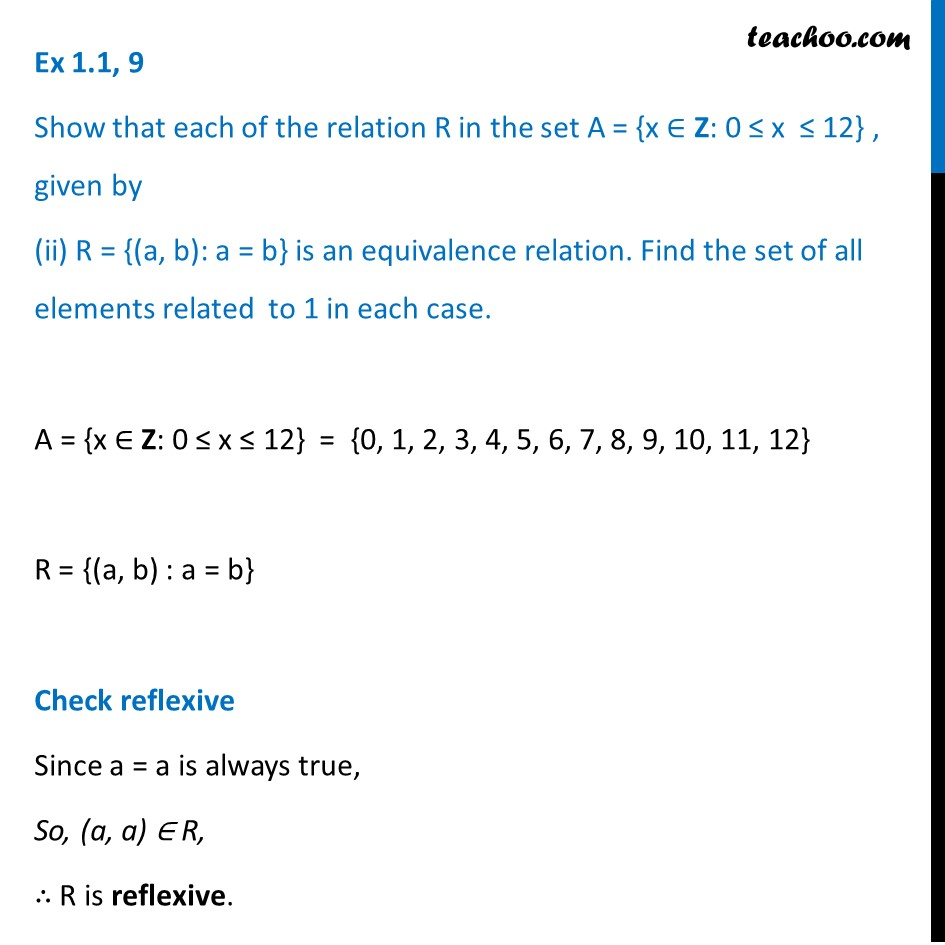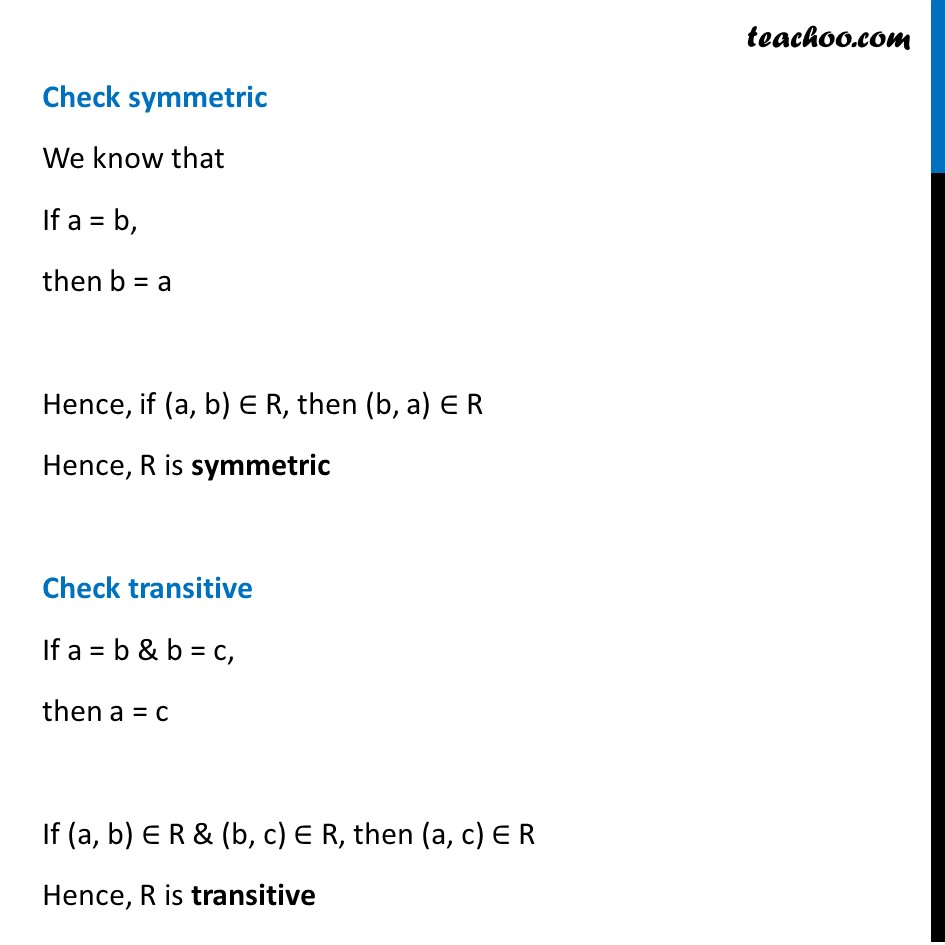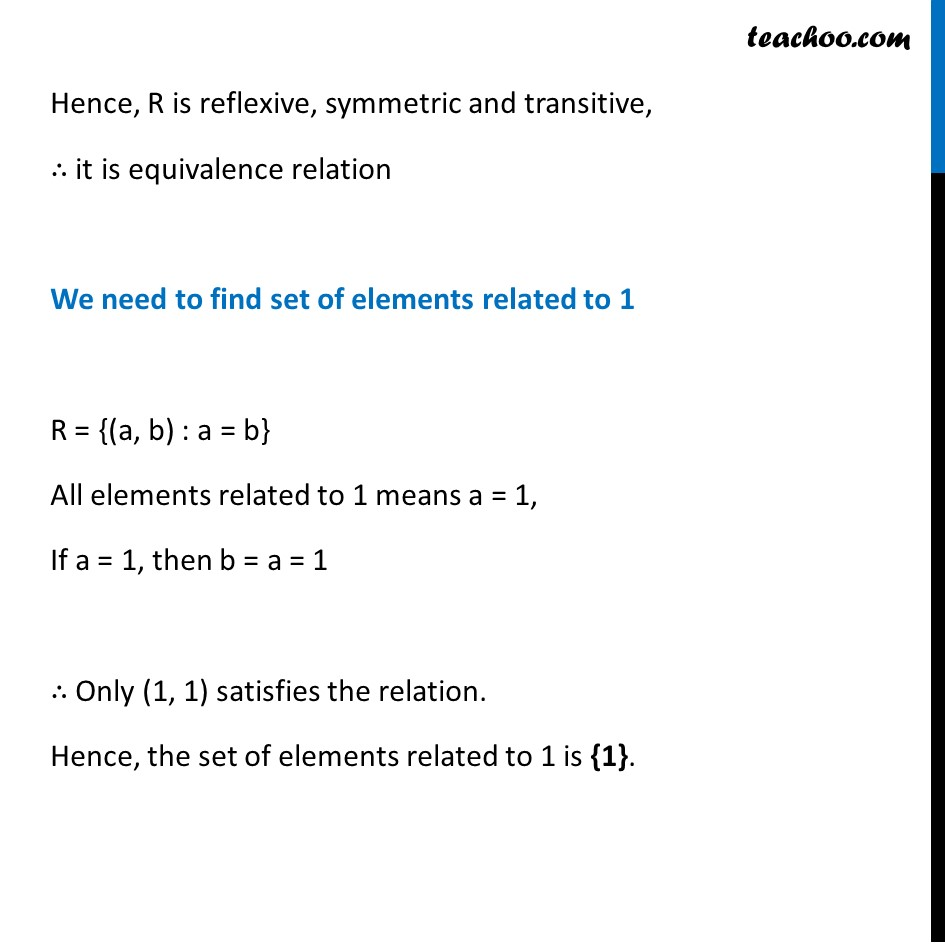Ex 1.1

Chapter 1 Class 12 Relation and Functions
Serial order wiseLearn in your speed, with individual attention - Teachoo Maths 1-on-1 Class

### Transcript

Ex 1.1, 9 Show that each of the relation R in the set A = {x ∈ Z: 0 ≤ x ≤ 12} , given by (ii) R = {(a, b): a = b} is an equivalence relation. Find the set of all elements related to 1 in each case. A = {x ∈ Z: 0 ≤ x ≤ 12} = {0, 1, 2, 3, 4, 5, 6, 7, 8, 9, 10, 11, 12} R = {(a, b) : a = b} Check reflexive Since a = a is always true, So, (a, a) ∈ R, ∴ R is reflexive. Check symmetric We know that If a = b, then b = a Hence, if (a, b) ∈ R, then (b, a) ∈ R Hence, R is symmetric Check transitive If a = b & b = c, then a = c If (a, b) ∈ R & (b, c) ∈ R, then (a, c) ∈ R Hence, R is transitive Hence, R is reflexive, symmetric and transitive, ∴ it is equivalence relation We need to find set of elements related to 1 R = {(a, b) : a = b} All elements related to 1 means a = 1, If a = 1, then b = a = 1 ∴ Only (1, 1) satisfies the relation. Hence, the set of elements related to 1 is {1}.